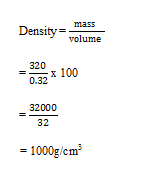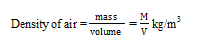# PHY1: DENSITY

##### This unit is about density in Physics and how it is measured.

Definition

Density is mass per unit volume.

In the laboratory experiments g/cm3 are normally used.

To obtain the density of a substance, we measure the mass and volume then calculate using the formula

Note:

For a particular substance, the density [ratio of mass to volume] is always constant is it does not change with the change in quantity.

EXAMPLES

Given that a mass of 320g of water occupies 0.32cm3. Calculate the density of this water.DENSITY OF LIQUIDS

Get the mass of the object first by;

– getting a container and beam balance

– get the mass of the container and note it down.

– pour the water into the container and place it on a beam balance and note the reading.

Subtract the mass of empty container from the one with water.

1. Then find the volume of the liquid by;-

-get a measuring cylinder pour in the liquid on flat surface and wait for the liquid to settle and read off.

1. Describe how you would determine the density of a solid object

– First dean the beam balance and the object to be measured

– Then place the object on the beam balance and record the mass.

– You get a measuring cylinder with water and record the first volume.

– Place in the object and measure the second volume.

– Then subtract the first volume from the second one. To get the one of the solid object [v2 –v1]

– Get the density by using the formula, mass divide by the volume.Since density may be given in g/cm3 or kg/m3, we may be required to convert from one unit to another.

EXAMPLES

Convert the following as required

Experiment to measure the density of dry air;-

A clean dry round bottomed flask is filled with rubber bang through which passes a short glass tube carrying a short length of rubber pressure tubing fitted with a screw clip.

With the clip open, the air is evacuated from the flask by connecting it to a good vacuum pump.

All the air will have been removed, when the levels are the same on both sides of the closed and the manometer, the clip is then closed and the mass of the flask measured with a balance.

The flask is now connected to a calcium chloride drying tube and the clip opened slowly to admit dry air into the flask.

When no more air enters, the clip is closed and the mass of the flask predetermined. The atmosphere pressure is read from a brome and the temperature is also noted.

The volume of the air is found by filling the flask with water and instating the bung with the clip open.

The bung is removed and the volume of the water found by using a suitable measuring cylinder. Record the results as below;

• In actual values instead of symbols

Temperature

Barometric pressure

Volume of air (v)

Mass of flask full of air (m2)

Mass of air = [m2 – m1] mgSTANDARD FORM

This is also known as scientific notation. Numbers are written in the form of a x 10n = where 1 a 10 and n is negative or positive integers.

EXAMPLES

1. 340= 0.34 x 10+3
1. 894 = 8.94 x  102
2. 0.00391 = 3.91 x 10-3
3. 401 = 4.01 x 102

THIS VIDEO EXPLAINS MORE ABOUT DENSITY

## STEM Elearning

We at FAWE have built this platform to aid learners, trainers and mentors get practical help with content, an interactive platform and tools to power their teaching and learning of STEM subjects, more